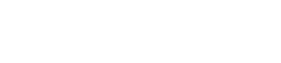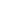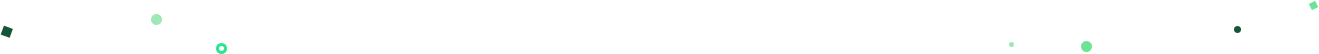NOW LIVEEmpower your end users with Explorations in Mode.Try it now## Mode Studio

The Collaborative Data Science Platform# SQL CASE

Starting here? This lesson is part of a full-length tutorial in using SQL for Data Analysis. Check out the beginning.

In this lesson we'll cover:

For the next few lessons, you'll work with data on College Football Players. This data was collected from ESPN on January 15, 2014 from the rosters listed on this page using a Python scraper available here. In this particular lesson, you'll stick to roster information. This table is pretty self-explanatory—one row per player, with columns that describe attributes for that player. Run this query to check out the raw data:

``````SELECT * FROM benn.college_football_players
``````

## The SQL CASE statement

The `CASE` statement is SQL's way of handling if/then logic. The `CASE` statement is followed by at least one pair of `WHEN` and `THEN` statements—SQL's equivalent of IF/THEN in Excel. Because of this pairing, you might be tempted to call this SQL `CASE WHEN`, but `CASE` is the accepted term.

Every `CASE` statement must end with the `END` statement. The `ELSE` statement is optional, and provides a way to capture values not specified in the `WHEN`/`THEN` statements. `CASE` is easiest to understand in the context of an example:

``````SELECT player_name,
year,
CASE WHEN year = 'SR' THEN 'yes'
ELSE NULL END AS is_a_senior
FROM benn.college_football_players
``````

In plain English, here's what's happening:

1. The `CASE` statement checks each row to see if the conditional statement—`year = 'SR'` is true.
2. For any given row, if that conditional statement is true, the word "yes" gets printed in the column that we have named `is_a_senior`.
3. In any row for which the conditional statement is false, nothing happens in that row, leaving a null value in the `is_a_senior` column.
4. At the same time all this is happening, SQL is retrieving and displaying all the values in the `player_name` and `year` columns.

The above query makes it pretty easy to see what's happening because we've included the `CASE` statement along with the `year` column itself. You can check each row to see whether `year` meets the condition `year = 'SR'` and then see the result in the column generated using the `CASE` statement.

But what if you don't want null values in the `is_a_senior` column? The following query replaces those nulls with "no":

``````SELECT player_name,
year,
CASE WHEN year = 'SR' THEN 'yes'
ELSE 'no' END AS is_a_senior
FROM benn.college_football_players
``````

## Practice Problem

Write a query that includes a column that is flagged "yes" when a player is from California, and sort the results with those players first.

Try it out See the answer

## Adding multiple conditions to a CASE statement

You can also define a number of outcomes in a `CASE` statement by including as many `WHEN`/`THEN` statements as you'd like:

``````SELECT player_name,
weight,
CASE WHEN weight > 250 THEN 'over 250'
WHEN weight > 200 THEN '201-250'
WHEN weight > 175 THEN '176-200'
ELSE '175 or under' END AS weight_group
FROM benn.college_football_players
``````

In the above example, the `WHEN`/`THEN` statements will get evaluated in the order that they're written. So if the value in the `weight` column of a given row is 300, it will produce a result of "over 250." Here's what happens if the value in the `weight` column is 180, SQL will do the following:

1. Check to see if `weight` is greater than 250. 180 is not greater than 250, so move on to the next `WHEN`/`THEN`
2. Check to see if `weight` is greater than 200. 180 is not greater than 200, so move on to the next `WHEN`/`THEN`
3. Check to see if `weight` is greater than 175. 180 is greater than 175, so record "175-200" in the `weight_group` column.

While the above works, it's really best practice to create statements that don't overlap. `WHEN weight > 250` and `WHEN weight > 200` overlap for every value greater than 250, which is a little confusing. A better way to write the above would be:

``````SELECT player_name,
weight,
CASE WHEN weight > 250 THEN 'over 250'
WHEN weight > 200 AND weight <= 250 THEN '201-250'
WHEN weight > 175 AND weight <= 200 THEN '176-200'
ELSE '175 or under' END AS weight_group
FROM benn.college_football_players
``````

## Practice Problem

Write a query that includes players' names and a column that classifies them into four categories based on height. Keep in mind that the answer we provide is only one of many possible answers, since you could divide players' heights in many ways.

Try it out See the answer

You can also string together multiple conditional statements with `AND` and `OR` the same way you might in a `WHERE` clause:

``````SELECT player_name,
CASE WHEN year = 'FR' AND position = 'WR' THEN 'frosh_wr'
ELSE NULL END AS sample_case_statement
FROM benn.college_football_players
``````

## A quick review of CASE basics:

1. The `CASE` statement always goes in the `SELECT` clause
2. `CASE` must include the following components: `WHEN`, `THEN`, and `END`. `ELSE` is an optional component.
3. You can make any conditional statement using any conditional operator (like `WHERE` ) between `WHEN` and `THEN`. This includes stringing together multiple conditional statements using `AND` and `OR`.
4. You can include multiple `WHEN` statements, as well as an `ELSE` statement to deal with any unaddressed conditions.

## Practice Problem

Write a query that selects all columns from `benn.college_football_players` and adds an additional column that displays the player's name if that player is a junior or senior.

Try it out See the answer

## Using CASE with aggregate functions

`CASE`'s slightly more complicated and substantially more useful functionality comes from pairing it with aggregate functions. For example, let's say you want to only count rows that fulfill a certain condition. Since `COUNT` ignores nulls, you could use a `CASE` statement to evaluate the condition and produce null or non-null values depending on the outcome:

``````SELECT CASE WHEN year = 'FR' THEN 'FR'
ELSE 'Not FR' END AS year_group,
COUNT(1) AS count
FROM benn.college_football_players
GROUP BY CASE WHEN year = 'FR' THEN 'FR'
ELSE 'Not FR' END
``````

Now, you might be thinking "why wouldn't I just use a `WHERE` clause to filter out the rows I don't want to count?" You could do that—it would look like this:

``````SELECT COUNT(1) AS fr_count
FROM benn.college_football_players
WHERE year = 'FR'
``````

But what if you also wanted to count a couple other conditions? Using the `WHERE` clause only allows you to count one condition. Here's an example of counting multiple conditions in one query:

``````SELECT CASE WHEN year = 'FR' THEN 'FR'
WHEN year = 'SO' THEN 'SO'
WHEN year = 'JR' THEN 'JR'
WHEN year = 'SR' THEN 'SR'
ELSE 'No Year Data' END AS year_group,
COUNT(1) AS count
FROM benn.college_football_players
GROUP BY 1
``````

The above query is an excellent place to use numbers instead of columns in the `GROUP BY` clause because repeating the `CASE` statement in the `GROUP BY` clause would make the query obnoxiously long. Alternatively, you can use the column's alias in the `GROUP BY` clause like this:

``````SELECT CASE WHEN year = 'FR' THEN 'FR'
WHEN year = 'SO' THEN 'SO'
WHEN year = 'JR' THEN 'JR'
WHEN year = 'SR' THEN 'SR'
ELSE 'No Year Data' END AS year_group,
COUNT(1) AS count
FROM benn.college_football_players
GROUP BY year_group
``````

Note that if you do choose to repeat the entire `CASE` statement, you should remove the `AS year_group` column naming when you copy/paste into the `GROUP BY` clause:

``````SELECT CASE WHEN year = 'FR' THEN 'FR'
WHEN year = 'SO' THEN 'SO'
WHEN year = 'JR' THEN 'JR'
WHEN year = 'SR' THEN 'SR'
ELSE 'No Year Data' END AS year_group,
COUNT(1) AS count
FROM benn.college_football_players
GROUP BY CASE WHEN year = 'FR' THEN 'FR'
WHEN year = 'SO' THEN 'SO'
WHEN year = 'JR' THEN 'JR'
WHEN year = 'SR' THEN 'SR'
ELSE 'No Year Data' END
``````

Combining `CASE` statements with aggregations can be tricky at first. It's often helpful to write a query containing the `CASE` statement first and run it on its own. Using the previous example, you might first write:

``````SELECT CASE WHEN year = 'FR' THEN 'FR'
WHEN year = 'SO' THEN 'SO'
WHEN year = 'JR' THEN 'JR'
WHEN year = 'SR' THEN 'SR'
ELSE 'No Year Data' END AS year_group,
*
FROM benn.college_football_players
``````

The above query will show all columns in the `benn.college_football_players` table, as well as a column showing the results of the `CASE` statement. From there, you can replace the `*` with an aggregation and add a `GROUP BY` clause. Try this process if you struggle with either of the following practice problems.

## Practice Problem

Write a query that counts the number of 300lb+ players for each of the following regions: West Coast (CA, OR, WA), Texas, and Other (Everywhere else).

Try it out See the answer

## Practice Problem

Write a query that calculates the combined weight of all underclass players (FR/SO) in California as well as the combined weight of all upperclass players (JR/SR) in California.

Try it out See the answer

## Using CASE inside of aggregate functions

In the previous examples, data was displayed vertically, but in some instances, you might want to show data horizontally. This is known as "pivoting" (like a pivot table in Excel). Let's take the following query:

``````SELECT CASE WHEN year = 'FR' THEN 'FR'
WHEN year = 'SO' THEN 'SO'
WHEN year = 'JR' THEN 'JR'
WHEN year = 'SR' THEN 'SR'
ELSE 'No Year Data' END AS year_group,
COUNT(1) AS count
FROM benn.college_football_players
GROUP BY 1
``````

And re-orient it horizontally:

``````SELECT COUNT(CASE WHEN year = 'FR' THEN 1 ELSE NULL END) AS fr_count,
COUNT(CASE WHEN year = 'SO' THEN 1 ELSE NULL END) AS so_count,
COUNT(CASE WHEN year = 'JR' THEN 1 ELSE NULL END) AS jr_count,
COUNT(CASE WHEN year = 'SR' THEN 1 ELSE NULL END) AS sr_count
FROM benn.college_football_players
``````

It's worth noting that going from horizontal to vertical orientation can be a substantially more difficult problem depending on the circumstances, and is covered in greater depth in a later lesson.

## Practice Problem

Write a query that displays the number of players in each state, with FR, SO, JR, and SR players in separate columns and another column for the total number of players. Order results such that states with the most players come first.

Try it out See the answer

## Practice Problem

Write a query that shows the number of players at schools with names that start with A through M, and the number at schools with names starting with N - Z.

Try it out See the answer

Next Lesson

SQL DISTINCT# Get our weekly data newsletter

Work-related distractions for every data enthusiast.